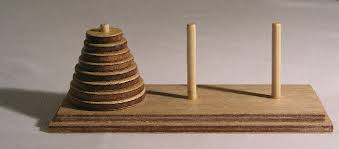# Hanoi wants more Towers

Probability Level 4The Tower of Hanoi is a traditional game where there are 3 pegs and $n$ discs arranged as seen in picture. All discs are to be moved from $1^{st}$ peg $P_1$ to $3^{rd}$ peg $P_3$ such that only one disc is moved at once. But no disc should be kept on one smaller than it during the process. So the minimum number of moves required to transfer all discs to $P_3$ (say $a_n$) is counted by $a_n=2a_{n-1}+1$ and $a_1=1$ i.e. after solving becomes $a_n=2^n-1.$

If we have a Tower of Hanoi with 42 pegs, and we have an additional rule that no disc can be moved more than twice, what is the maximum number of discs such that all discs can be moved to pegs other than the first peg $P_1?$

×